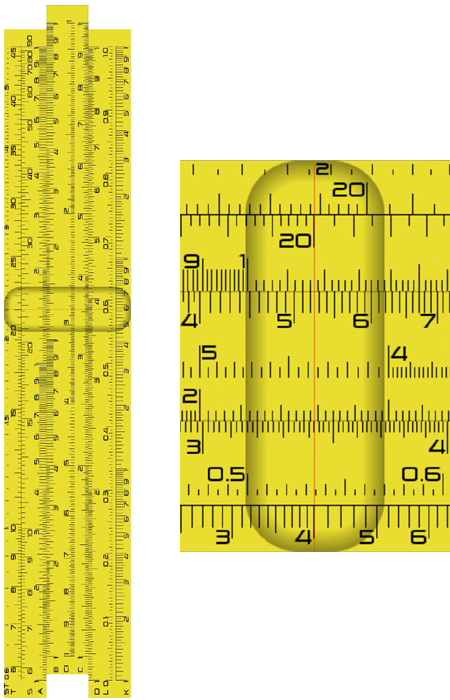# Definition: slide rule

An early computing device invented by Reverend William Oughtred in London in the 17th century. Primarily for multiplication and division, the slide rule has two stationary sets and one sliding set of numbers in the center. To multiply, numbers are added; to divide, numbers are subtracted. Subsequent slide rules added scales for trigonometric, powers and root computations.Slide Rule and Cursor
The vertical hairline on the movable "cursor" enables precise viewing. Because the slide rule is based on a logarithmic scale, the spacing between numerical divisions decreases as the values increase. These examples were taken from the Android Slide Rule app from Gushiku Studios LLC.Multiply 2 Times 3
The 1 on the C scale is set to 2 on the D scale. Then the cursor is moved to 3 on the C scale, and the result is read on the D scale.